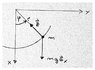# Pendulum and constraining forces (Lagrangian mechanics)

• JulienB
In summary, the masspoint moves on a circular path under the influence of gravity, but must additionally maintain its position by applying a force in the radial direction. The equation of motion for the masspoint can be written as a function of only the Lagrange function and the potential energy.

## Homework Statement

Hi everybody! As always, I struggle with my special relativity class and here is a new problem I'd like to have some indications about:

A masspoint m moves in the x-y-plane under the influence of gravity on a circular path of radius r (see attached pic). Which constraining force ##\vec{z}## must be additionally be acting in the radial direction, so that the masspoint remains on the circular path? You must first establish the equation of motion for the masspoint m and integrate it for the case small angle ##\varphi##.

## Homework Equations

Lagrangian mechanics, holonomic constraints

## The Attempt at a Solution

So first I wrote the coordinates dependently of each other and in function of ##l##:

##x^2 + y^2 = l^2 \iff x^2 + y^2 - l^2 = 0##

If I understand my script correctly, such an equation ##f(x,y,l,t) = 0## means this is a case of holonomic constraint. Right? I am not sure if ##l## should be included in my function. Anyway I can rewrite ##x## and ##y## as

##x = l \cdot \cos \varphi## and ##y = l \ cdot \sin \varphi##
##\implies \dot{x} = - \varphi l \sin \varphi## and ##\dot{y} = \varphi l \cos \varphi##

I can now substitute those values in the Lagrange function to have an equation of motion only depending on ##l## and ##\varphi##:

##L = T - V = \frac{1}{2} m (\dot{x}^2 + \dot{y}^2) - mgh##
## = \frac{1}{2} m\varphi^2 l^2 (\cos^2\varphi + \sin^2\varphi) - mgh##
## = \frac{1}{2} m \varphi^2 l^2 - mgh##

That went pretty well until now, but now I'm blocked because of the ##h##. How can I express it in terms of ##l## and ##\varphi##? Geometrically I see the relation but I don't know what ##h## is. Anything I am missing?

Julien.

#### Attachments

•Photo 12-05-16 18 36 03.jpeg
136.1 KB · Views: 486
Wouldn't ##\left (1 - \cos\phi\right)## qualify for the job ?

@BvU You mean for ##h##? Did you see the picture? I searched a bit on the internet, and everyone seems to use a different coordinate system. For example in page 5 of this link (http://www.math.pitt.edu/~bard/classes/1270/mechanics.pdf) is ##h=-l\cos \varphi## apparently.

(Note that my teacher has inversed the ##x## and ##y## axes, probably to make it just confusing enough so that we don't get it.. I'm unsure why is ##y = -l \cos \varphi## in the link I put in post #3 and not ##l \cos \varphi## as for my ##x##. Is that just because my ##x## axis points downwards?)

I think I got it, I must have misunderstood something about the potential. Apparently I can just define a zero potential energy point which conveniently would be ##\varphi = 0##, then my potential would be as said @BvU ##V = -mg\Delta h = -mg l (1 - cos \varphi)##. Is that correct?

JulienB said:
@BvU You mean for ##h##? Did you see the picture? I searched a bit on the internet, and everyone seems to use a different coordinate system. For example in page 5 of this link (http://www.math.pitt.edu/~bard/classes/1270/mechanics.pdf) is ##h=-l\cos \varphi## apparently.
Yes, potential can be defined wrt an arbitrary point.
And x/y are now out of the picture with this generalized coordinate, so which way they point isn't all that relevant anymore. But I agree the choice is somewhat unusual.

@BvU Okay thanks a lot :) Then I get an equation of motion ##m \ddot{\varphi} = \frac{-m g \cdot \varphi}{l}##. If I am not mistaken, one can find the constraint force from the equation of motion with ##\sum \vec{F} = \vec{F_G} + \vec{Z}##, right?

Does that mean ##\vec{Z} = \frac{-m g \cdot \varphi}{l} - mg##?

Thanks a lot.

Julien.

Last edited: